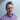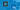Carl Rippon

# React Generic Props

April 28, 2020
typescriptreact

In a previous post, we explored different ways that React component props can be strongly-typed with TypeScript. In this post, we’ll cover a more advanced approach, which is to use a generic type for the props.

## Use cases

Generic props are useful when you want to create a reusable data-driven component where the shape of the data varies. Reusable form, list, and table components are examples where generic props are handy. In this post, we’ll create a simple reusable table component.

## Syntax

The syntax for the generic props type is as follows:

``type Props<T> = { ... };``

`T` is a parameter for the type of the data that the consumer can pass in. We’ll use a more meaningful name rather than T when we implement our example.

If we want to use an interface for the type then the syntax will be as follows:

``interface Props<T> { ... }``

The more tricky bit is applying this type to a React component:

``const ReusableComponent = <T extends object>(props: Props<T>) => { ... }``

The above syntax is for an arrow function component. Below is the syntax for a normal function component declaration:

``function ReusableComponent<T extends object>(props: Props<T>) { ... }``

We define the type that the consumer can pass into the component before the function parameter parentheses. Notice that we have used `T extends object` rather than just `T` otherwise we get a parsing error (we could also have used `<T,>` to avoid the parsing error).

If we want the data to have a particular property, then we can specify this in place of `object`. The example below requires the data to have a `name` property:

``<T extends {name: string}>``

Now that we are starting to understand the syntax, let’s step through an example use case.

## Generic table component props

We are going to implement a generic table component. Here’s our generic props type:

``````type Props<DataItem> = {
data: DataItem[];
onRowClick: (item: DataItem) => void;
};``````

We’ve chosen to name the type parameter `DataItem` which is the type of each item in the data. The props contain a `data` prop, which is an array of the data items. The props also contain an event prop, `onRowClick`. This will be invoked when a row in the table is clicked with the item of data for the row passed into it.

Below is the start of the table component implementation:

``const Table = <T extends object>({ data, onRowClick }: Props<T>) => {}``

We destructured the `data` and `onRowClick` props so that we can reference them directly in our implementation.

Our table component can be consumed as follows:

``````<Table
data={[
{ name: "Bill", score: 15 },
{ name: "Jane", score: 18 },
{ name: "Fred", score: 10 }
]}
onRowClick={row => console.log(row)}
/>``````

Notice that we don’t need to pass the component the type for the data because TypeScript cleverly infers it.

## Rendering the table column headings

We’re going to render the column headings in the table. Before we do that we are going to check whether there is any data and if not, we are going to render nothing:

``````const Table = <DataItem extends object>({ data, onRowClick }: Props<DataItem>) => {
if (data.length === 0) {    return null;  }};``````

Now let’s render the column headings:

``````const Table = <DataItem extends object>({ data, onRowClick }: Props<DataItem>) => {
if (data.length === 0) {
return null;
}
return (    <table>      <thead>        <tr>          {Object.keys(data).map(key => (            <th key={key}>{key}</th>          ))}        </tr>      </thead>    </table>  );};``````

We use `Object.keys` to get all the properties in the data and iterate through them with the `map` method outputting `th` element.

## Rendering the table data

Let’s move on to render the data in the table:

``````const Table = <T extends object>({ data, onRowClick }: Props<T>) => {
...
return (
<table>
...
<tbody>        {data.map((item: DataItem, index: number) => (          <tr key={index}>            {(Object.keys(data) as Array<keyof DataItem>).map(key => (              <td key={key.toString()}>{item[key]}</td>            ))}          </tr>        ))}      </tbody>    </table>
);
};``````

Notice that we have to use a type assertion on the result of `Object.keys` because by default it will return `string[]` which term would mean that `item[key]` raises a type error.

## Implementing the row click

The last step is to implement the row click:

``````<tbody>
{data.map((item: DataItem, index: number) => (
<tr key={index} onClick={() => onRowClick(item)}>        {(Object.keys(data) as Array<keyof DataItem>).map(key => (
<td key={key.toString()}>{item[key]}</td>
))}
</tr>
))}
</tbody>``````

Let’s check what the type of the `onRowClick` parameter has been inferred as in our consumer:

Neat!

This shows the power of generic props because even though the type of the data passed in can vary, the props are still strongly-typed for the consumer.

Here’s a link to this example in CodeSandbox.

## Wrap up

Generic props allow us to implement generic components that deal with varying shapes of data without compromising type safety. The syntax may be a bit weird a first, but it makes perfect sense after a while, and the result is well worth it.